• 风险矩阵图示 5乘5维度分析.pptx,pptfans_97e6a2cd49cc6951.pptx
• ## 多维度产品分析

千次阅读 2018-05-20 21:18:22
是一个多维度的产品分析。 产品有哪些基本要求呢？可以看到首先一个产品的存在是有价值的，无论这个产品是有形的实体还是无形的服务。它的价值可以体现在能够满足用户的需求，在这个基础上建立一系列的功能组合...
    下图是一个多维度的产品分析。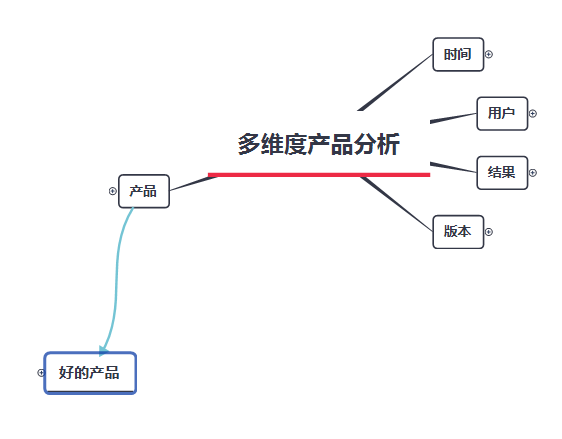产品有哪些基本要求呢？可以看到首先一个产品的存在是有价值的，无论这个产品是有形的实体还是无形的服务。它的价值可以体现在能够满足用户的需求，在这个基础上建立一系列的功能组合，创造良好的用户体验，保证其可用性（可行性前提）。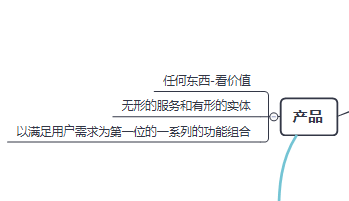那么一个好的产品还要具备哪些要素呢？如图，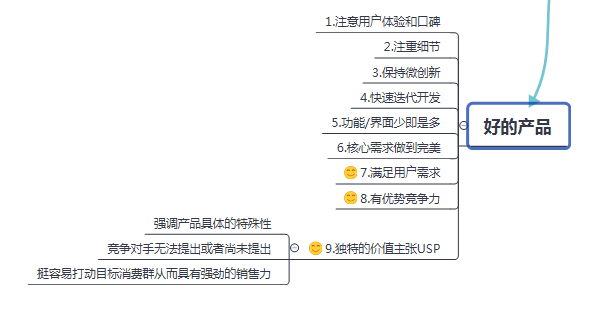关于生命周期，有些产品的成长期和成熟期很长，有些产品则很快就进入衰退期，产品经理要判断产品状态，就是判断一个产品是否能够继续做一些调整而延长成熟期甚至是进入成长期，或者直接的放弃。在产品的不同生命周期内，产品经理要运用不同的运营方式来推动产品的发展。
用户方面，大都数来讲，我们当初设定的目标用户很有可能不是我们产品使用最多的用户，目标用户可能只占20%，反而从众用户蛮多的。不过这都没有很大的关系，产品经理需要用16%的尝鲜者来带领68%的主流用户，挖掘那些有潜在价值的用户。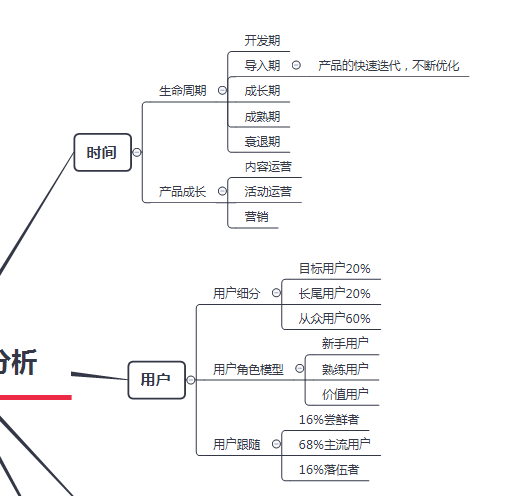最后是关于版本和结果的一些分类。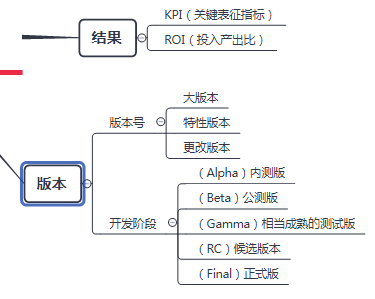展开全文• 一个维度的多角度对比分析 dts ("20050101", '%Y%m%d') + seq(0,1000,15) A ( Dates = dts, Gas = 4000 + cumsum(abs( rnorm(length(dts), 100, 30)))) head(A) A ( A, Year = format(Dates, '%Y'), Day...
R实战系列专栏
介绍
ggplot2是我见过最human friendly的画图软件，这得益于Leland Wilkinson在他的著作《The Grammar of Graphics》中提出了一套图形语法，把图形元素抽象成可以自由组合的成分，Hadley Wickham把这套想法在R中实现。

ggplot2基本要素
数据（Data）和映射（Mapping） 几何对象（Geometric） 标尺（Scale） 统计变换（Statistics） 坐标系统（Coordinante） 图层（Layer） 分面（Facet） 主题（Theme）
绘图
下面以一份钻石的数据为例，这份数据非常大，随机取一个子集来画图。

require(ggplot2)
data(diamonds)
set.seed(42)
small <- diamonds[sample(nrow(diamonds), 1000), ]
head(small)
> head(small)
carat       cut color clarity depth table price    x    y    z
49345  0.71 Very Good     H     SI1  62.5    60  2096 5.68 5.75 3.57
50545  0.79   Premium     H     SI1  61.8    59  2275 5.97 5.91 3.67
15434  1.03     Ideal     F     SI1  62.4    57  6178 6.48 6.44 4.03
44792  0.50     Ideal     E     VS2  62.2    54  1624 5.08 5.11 3.17
34614  0.27     Ideal     E     VS1  61.6    56   470 4.14 4.17 2.56
27998  0.30   Premium     E     VS2  61.7    58   658 4.32 4.34 2.67画图实际上是把数据中的变量映射到图形属性上。以克拉(carat)数为X轴变量，价格(price)为Y轴变量。

require(ggplot2)
data(diamonds)
set.seed(42)
small <- diamonds[sample(nrow(diamonds), 1000), ]#随机抽取1000个作为样本
#画图实际上是把数据中的变量映射到图形属性上。
#以克拉(carat)数为X轴变量，价格(price)为Y轴变量。
p <- ggplot(data = small, mapping = aes(x = carat, y = price))
#上面这行代码把数据映射XY坐标轴上，需要告诉ggplot2，这些数据要映射成什么样的几何对象，
#下面以散点为例：
p + geom_point()
#如果想将切工（cut）映射到形状属性。只需要：
p <- ggplot(data=small, mapping=aes(x=carat, y=price, shape=cut))
p + geom_point()
#再比如我想将钻石的颜色（color）映射颜色属性：
p <- ggplot(data=small, mapping=aes(x=carat, y=price, shape=cut, colour=color))
p+geom_point()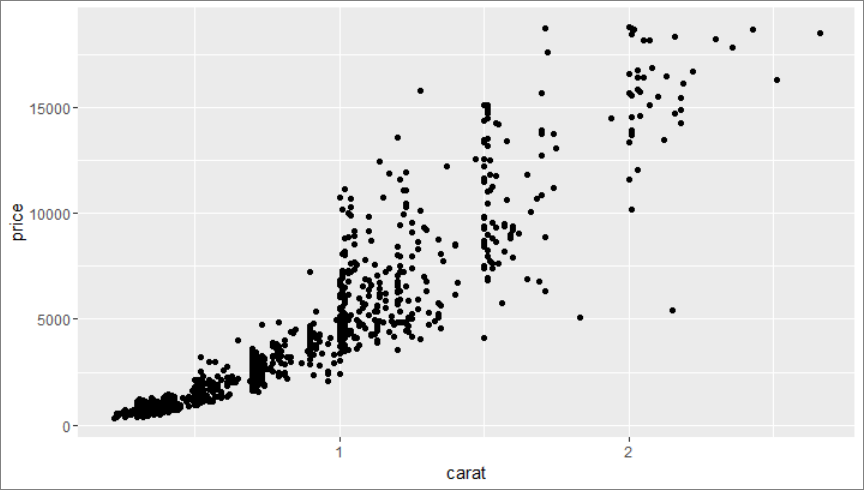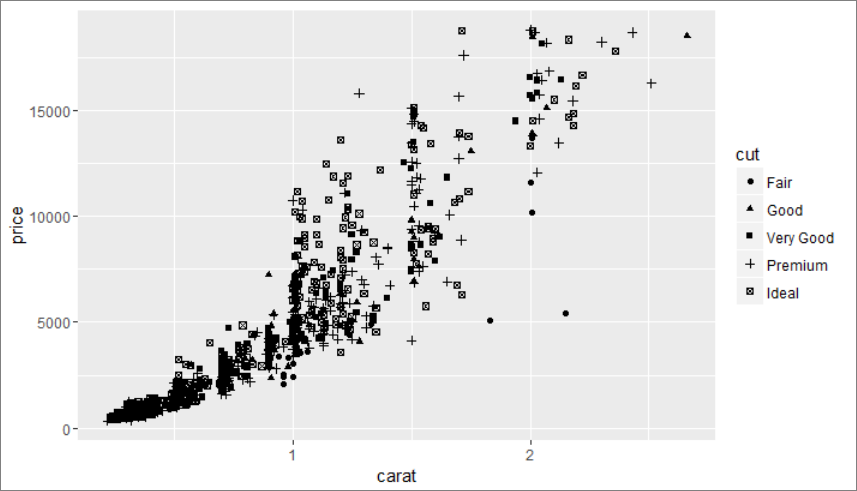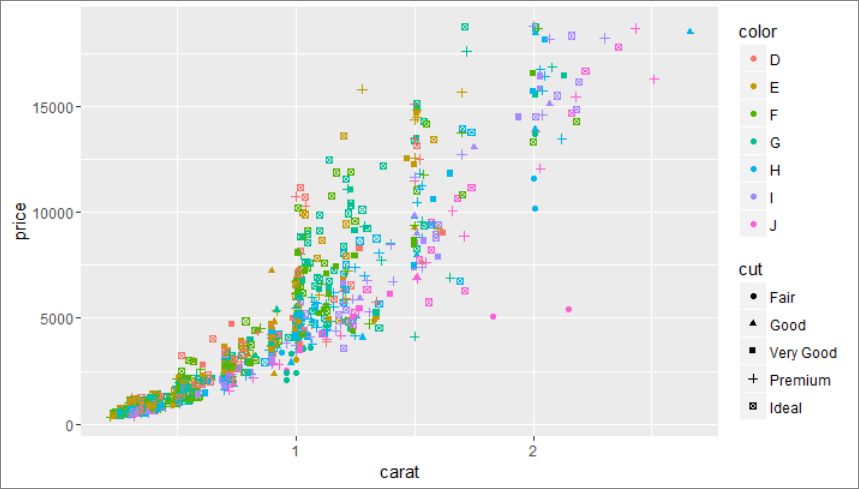更直观的绘图语法
最后一幅图也可以用下面的写法来完成
#再比如我想将钻石的颜色（color）映射颜色属性：
ggplot(data=small) + geom_point(aes(x=carat, y=price, shape=cut, colour=color))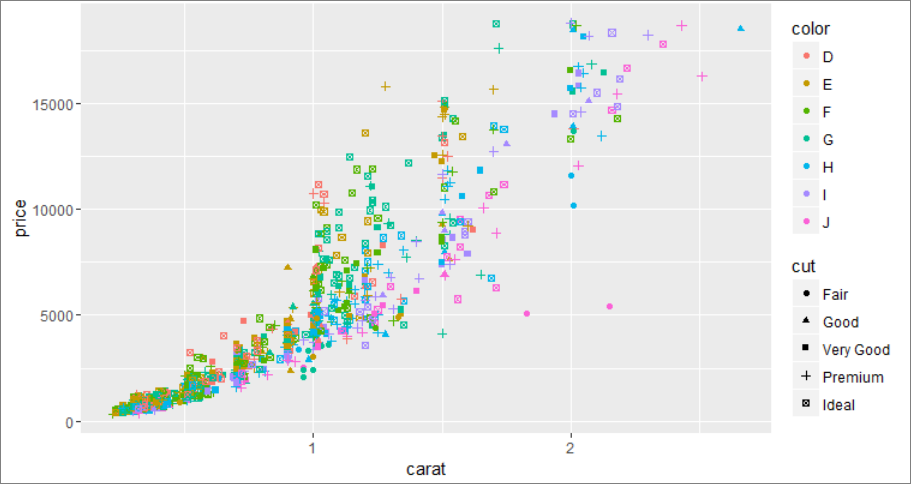分面(将一个维度拆分成多图)

p <- ggplot(mpg, aes(displ, cty)) + geom_point()
tail(mpg)
p + facet_grid(. ~ cyl)#将cyl维度拆分成：左右多图
p + facet_grid(cyl~.)#将cyl维度拆分成：上下多图
p + facet_grid(drv ~ .)#将drv维度拆分成：上下多图
p + facet_grid(drv ~ cyl)#将drv和cyl拆分成二维多图
> head(mpg)
manufacturer model displ year cyl      trans drv cty hwy fl   class
1         audi    a4   1.8 1999   4   auto(l5)   f  18  29  p compact
2         audi    a4   1.8 1999   4 manual(m5)   f  21  29  p compact
3         audi    a4   2.0 2008   4 manual(m6)   f  20  31  p compact
4         audi    a4   2.0 2008   4   auto(av)   f  21  30  p compact
5         audi    a4   2.8 1999   6   auto(l5)   f  16  26  p compact
6         audi    a4   2.8 1999   6 manual(m5)   f  18  26  p compact
> tail(mpg)
manufacturer  model displ year cyl      trans drv cty hwy fl   class
229   volkswagen passat   1.8 1999   4   auto(l5)   f  18  29  p midsize
230   volkswagen passat   2.0 2008   4   auto(s6)   f  19  28  p midsize
231   volkswagen passat   2.0 2008   4 manual(m6)   f  21  29  p midsize
232   volkswagen passat   2.8 1999   6   auto(l5)   f  16  26  p midsize
233   volkswagen passat   2.8 1999   6 manual(m5)   f  18  26  p midsize
234   volkswagen passat   3.6 2008   6   auto(s6)   f  17  26  p midsize
p + facet_grid(. ~ cyl)#将cyl维度拆分成：左右多图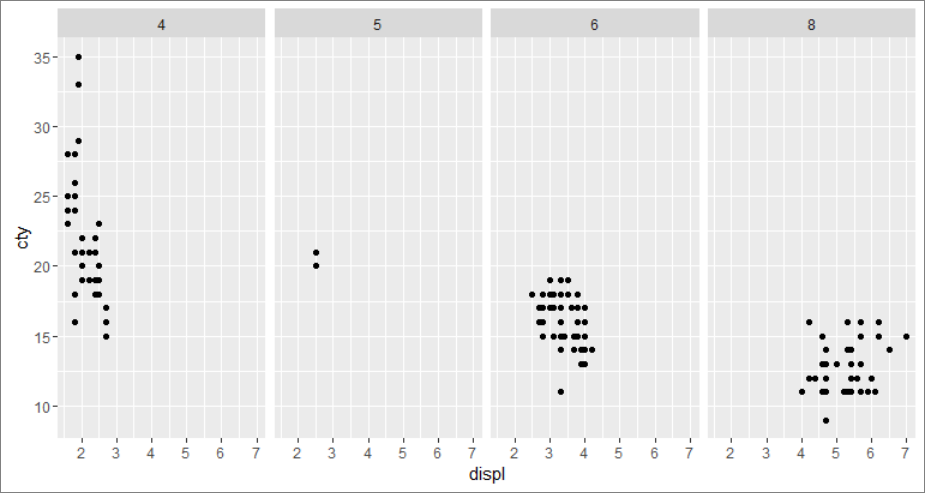p + facet_grid(cyl~.)#将cyl维度拆分成：上下多图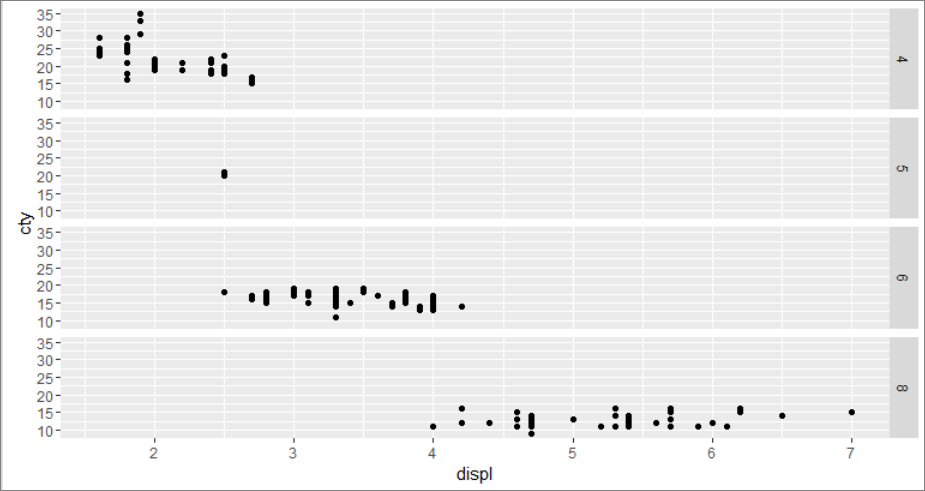p + facet_grid(drv ~ .)#将drv维度拆分成：上下多图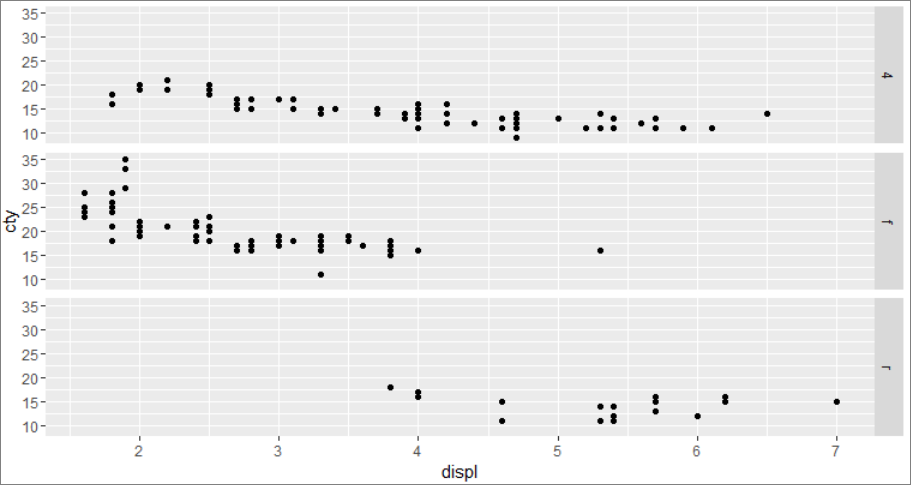p + facet_grid(drv ~ cyl)#将drv和cyl拆分成二维多图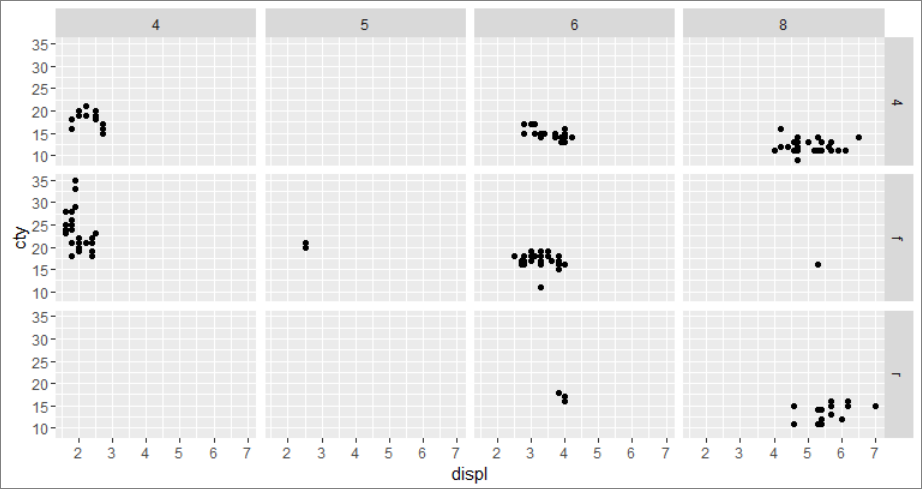一个维度的多角度对比分析

dts <- as.Date("20050101", '%Y%m%d') + seq(0,1000,15)
A <- data.frame( Dates = dts, Gas = 4000 + cumsum(abs( rnorm(length(dts), 100, 30))))
A <- transform( A,
Year = format(Dates, '%Y'),
DayOfYear = as.numeric( format(Dates, '%j')),
GasDiff = c(diff( Gas ),NA))
require(ggplot2)
ggplot(A, aes(Dates, GasDiff)) + geom_line( aes(colour = Year))#不同的年份不同的颜色
ggplot(A, aes(DayOfYear, GasDiff)) + geom_line( )  + facet_grid(Year ~ .)#不同的年份不同的图框(上下放置)
ggplot(A, aes(DayOfYear, GasDiff)) + geom_line( aes(colour = Year) )#不同的年份不同颜色(不拆分图框)
ggplot(A, aes(DayOfYear, GasDiff)) + geom_line( aes(colour = Year) )  + geom_point( aes(shape = Year))#不同的年份不同颜色(不拆分图框)


> head(A)
Dates      Gas
1 2005-01-01 4149.130
2 2005-01-16 4285.929
3 2005-01-31 4371.898
4 2005-02-15 4451.551
5 2005-03-02 4602.443
6 2005-03-17 4748.729

> A <- transform( A,
+                 Year = format(Dates, '%Y'),
+                 DayOfYear = as.numeric( format(Dates, '%j')),
+    .... [TRUNCATED]

Dates      Gas Year DayOfYear   GasDiff
1 2005-01-01 4149.130 2005         1 136.79945
2 2005-01-16 4285.929 2005        16  85.96883
3 2005-01-31 4371.898 2005        31  79.65307
4 2005-02-15 4451.551 2005        46 150.89207
5 2005-03-02 4602.443 2005        61 146.28541
6 2005-03-17 4748.729 2005        76 106.43583ggplot(A, aes(Dates, GasDiff)) + geom_line( aes(colour = Year))#不同的年份不同的颜色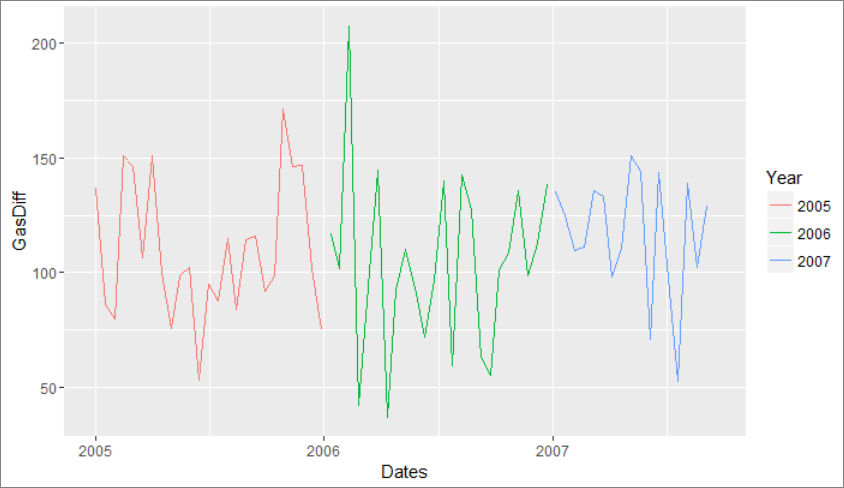ggplot(A, aes(DayOfYear, GasDiff)) + geom_line( )  + facet_grid(Year ~ .)#不同的年份不同的图框(上下放置)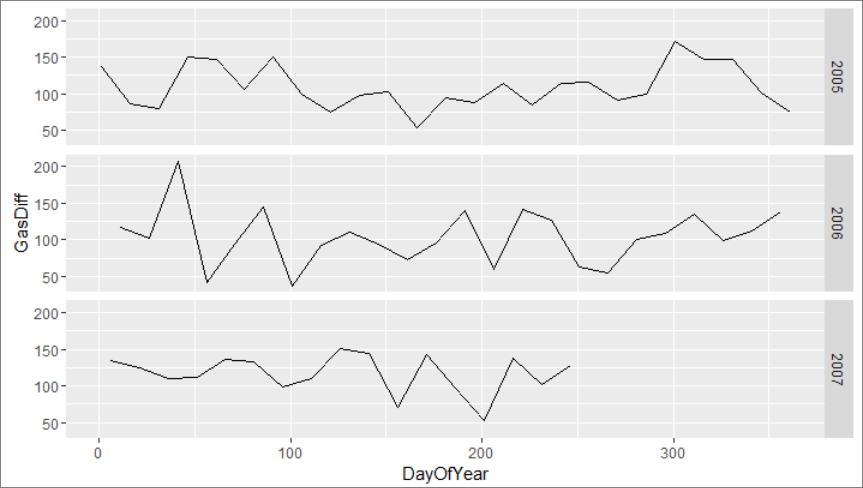ggplot(A, aes(DayOfYear, GasDiff)) + geom_line( aes(colour = Year) )#不同的年份不同颜色(不拆分图框)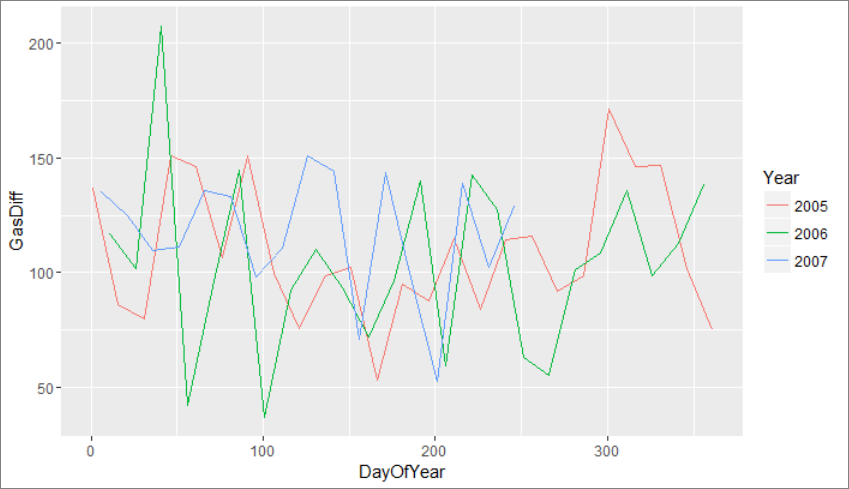ggplot(A, aes(DayOfYear, GasDiff)) + geom_line( aes(colour = Year) )  + geom_point( aes(shape = Year))#不同的年份不同颜色(不拆分图框)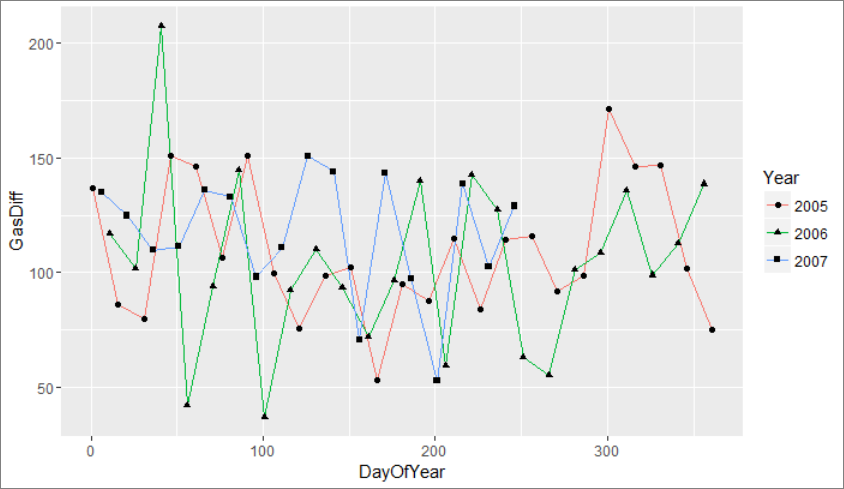参考：https://www.plob.org/article/7264.html
展开全文万次阅读 多人点赞 2019-09-29 11:04:41


文章目录Q

∈

R

(

N

,

T

q

,

d

m

o

d

e

l

)

Q \in \mathbb R^{(N,T_q, d_{model})}

,

K

∈

R

(

N

,

T

k

,

d

m

o

d

e

l

)

K \in \mathbb R^{(N,T_k,d_{model})}

,

V

∈

R

(

N

,

T

k

,

d

m

o

d

e

l

)

V \in \mathbb R^{(N,T_k,d_{model})}

。其中

N

N

是batch_size，

T

q

T_q

时

Q

Q

的maxlen，

T

k

T_k

是

K

K

的maxlen，

d

m

o

d

e

l

d_{model}

是最初单个词embedding的向量长度  接下来进行的是

h

h

次线性变换，

h

h

实际就是多头注意力的头数，假设第

i

i

次线性变换后会得到

Q

i

∗

,

K

i

∗

,

V

i

∗

Q^*_i,K^*_i,V^*_i

，变换方式如下：

Q

i

∗

=

Q

W

i

Q

W

i

Q

∈

R

(

d

m

o

d

e

l

,

d

k

)

K

i

∗

=

K

W

i

K

W

i

K

∈

R

(

d

m

o

d

e

l

,

d

k

)

V

i

∗

=

V

W

i

V

W

i

V

∈

R

(

d

m

o

d

e

l

,

d

v

)

Q^*_i = QW^Q_i \qquad W^Q_i \in \mathbb R^{(d_{model}, d_k)}\\ \\ K^*_i = KW^K_i \qquad W^K_i \in \mathbb R^{(d_{model}, d_k)}\\ \\ V^*_i = VW^V_i \qquad W^V_i \in \mathbb R^{(d_{model}, d_v)}\\ \\

因此，

Q

i

∗

∈

R

(

N

,

T

q

,

d

k

)

Q^*_i \in \mathbb R^{(N,T_q,d_k)}

,

K

i

∗

∈

R

(

N

,

T

k

,

d

k

)

K^*_i \in \mathbb R^{(N,T_k,d_k)}

,

V

i

∗

∈

R

(

N

,

T

k

,

d

v

)

V^*_i \in \mathbb R^{(N,T_k,d_v)}

接下来将

Q

i

∗

,

K

i

∗

,

V

i

∗

Q^*_i,K^*_i,V^*_i

进行attention的操作我们分别来看：

t

e

m

p

1

=

Q

i

∗

(

K

i

∗

)

T

d

k

t

e

m

p

1

∈

R

(

N

,

T

q

,

T

k

)

h

e

a

d

i

=

a

t

t

e

n

t

i

o

n

(

Q

i

∗

,

K

i

∗

,

V

i

∗

)

=

s

o

f

t

m

a

x

(

t

e

m

p

)

V

temp_1 = \frac {Q^*_i(K^*_i)^T}{\sqrt d_k} \qquad temp_1 \in \mathbb R^{(N,T_q,T_k)}\\ head_i = attention(Q^*_i, K^*_i,V^*_i) = softmax(temp)V

因此我们可以看出来

h

e

a

d

i

∈

R

(

N

,

T

q

,

d

v

)

head_i \in \mathbb R^{(N, T_q, d_v)}

然后我们进行的操作是，将

h

h

次attention得到的操作连接起来，并乘

W

O

W^O

矩阵

t

e

m

p

2

=

c

o

n

c

a

t

(

(

h

e

a

d

1

,

h

e

a

d

2

,

.

.

.

.

.

.

,

h

e

a

d

h

)

,

−

1

)

t

e

m

p

2

∈

R

(

N

,

T

q

,

h

d

v

)

M

u

l

t

i

−

H

e

a

d

(

Q

,

K

,

V

)

=

t

e

m

p

2

W

O

W

O

∈

R

(

N

,

h

d

v

,

d

m

o

d

e

l

)

因此最终得到的结果的维度是

R

(

N

,

T

q

,

d

m

o

d

e

l

)

\mathbb R^{(N, T_q, d_{model})}

。你应该会发现，和原本输入的

Q

Q

是同样的维度

Transfromer的训练过程
我们首先做一个假设，训练的batch大小为1,暂时忽略掉Positional Encoding
一个batch包含代翻译的句子集

X

X

和翻译后的句子集（ground_truth）

Y

Y

，其中:

X

∈

R

(

1

,

T

1

,

d

m

o

d

e

l

)

Y

∈

R

(

1

,

T

2

,

d

m

o

d

e

l

)

X \in \mathbb R^{(1, T_1,d_{model})} \\ Y \in \mathbb R^{(1, T_2,d_{model})} \\

T

1

T_1

是代翻译句子的最大长度，

T

2

T_2

是ground_truth的最大长度联系上图，训练过程的encoder下方的Inputs是

X

X

,decoder下方的Outputs是

Y

Y

因为Feed Forward层不会改变矩阵维度，所以encoder的输出的向量空间其实就是

R

(

1

,

T

1

,

d

m

o

d

e

l

)

\mathbb R^{(1, T_1, d_{model})}

R

(

1

,

T

2

,

d

m

o

d

e

l

)

\mathbb R^{(1,T_2,d_{model})}

decoder的第二层attention并非self-attention，encoder的输出作为

K

K

和

V

V

R

(

1

,

T

2

,

d

m

o

d

e

l

)

\mathbb R^{(1,T_2,d_{model})}

上述的linear和softmax操作最终将矩阵变为维度为

(

1

,

T

2

,

v

o

c

a

b

_

s

i

z

e

)

(1,T_2,vocab\_size)

，vocab_size是翻译的目标语言词表大小
最终softmax输出的矩阵维度是

(

1

,

T

2

,

v

o

c

a

b

_

s

i

z

e

)

(1,T_2,vocab\_size)

，

T

2

T_2

表示的是groud_truth的maxlen，因此矩阵第二维的每一个向量都代表着这个句子中一个词的概率分布，比如

（

1

，

2

，

3

）

（1，2，3）

代表的是第一个句子的第二个词是词表第三个词的概率
由上也不难理解为啥transformer的训练过程可以并行
因此transformer其实是对groud_truth的每一个词进一次预测，所以其损失函数就可以是交叉熵函数

Transformer的句子生成过程对于测试样例或者没有ground_truth的样本，Transformer生成句子的模式就和上图差不多(真实的Transformer是由6个encoder和6个decoder组成的)
每一个词的生成都需要输入之前生成的内容，这也是为什么测试和评估过程不是并行的原因

我们依次来进行介绍，在此之前我们进行一个假设

T

1

=

4

,

T

2

=

4

,

d

k

=

4

,

d

v

=

4

T_1=4,T_2=4,d_k=4,d_v=4

我们来看一个维度和单个句子一样的矩阵

[

1

1

1

1

1

1

1

1

0

0

0

0

0

0

0

0

]

\begin{bmatrix} 1&1&1&1 \\ 1&1&1&1 \\ 0&0&0&0 \\ 0&0&0&0 \end{bmatrix}

[

1

1

0

0

1

1

0

0

1

1

0

0

1

1

0

0

]

\begin{bmatrix} 1&1&0&0\\ 1&1&0&0\\ 1&1&0&0\\ 1&1&0&0\\ \end{bmatrix}

Q

K

T

QK^T

计算之后，对

Q

K

T

QK^T

K

K

经过了转置，所以相应的0的位置也变到了列上面来，于是将

Q

K

T

QK^T

的响应位置替换为负的很大值，那么

s

o

f

t

m

a

x

(

Q

K

T

)

softmax(QK^T)

对应位置的attention权重就会接近0
padding_num = -2 ** 32 + 1
# ones_like表示的是创建一个同样维度全都是1的矩阵
# tf.where(input, a, b)表示a中input对应位置维0的不变，其余的替换为b位置的数值


Q

Q

masks = tf.sign(tf.reduce_sum(tf.abs(queries), axis=-1))  # (N, T_q)


[

1

0

0

0

1

1

0

0

1

1

1

0

1

1

1

1

]

\begin{bmatrix} 1&0&0&0\\ 1&1&0&0\\ 1&1&1&0\\ 1&1&1&1\\ \end{bmatrix}

这个是在

Q

K

T

QK^T

之后，softmax之前的进行的，我们来慢慢分析

Q

=

K

=

V

Q=K=V

,我们假设句子是这样的(其中

s

1

,

s

2

,

s

3

,

s

4

s_1,s_2,s_3,s_4

都是词向量，并假设

T

1

=

T

2

=

4

T_1=T_2=4

)

Q

=

K

=

V

=

[

s

1

s

2

s

3

s

4

]

Q = K = V = \begin{bmatrix} s_1\\s_2\\s_3\\s_4 \end{bmatrix}

接下来进行计算

Q

K

T

QK^T

Q

K

T

=

[

s

1

s

1

T

s

1

s

2

T

s

1

s

3

T

s

1

s

4

T

s

2

s

1

T

s

2

s

2

T

s

2

s

3

T

s

2

s

4

T

s

3

s

1

T

s

3

s

2

T

s

3

s

3

T

s

3

s

4

T

s

4

s

1

T

s

4

s

2

T

s

4

s

3

T

s

4

s

4

T

]

QK^T=\begin{bmatrix} s_1s_1^T&s_1s_2^T&s_1s_3^T&s_1s_4^T \\ s_2s_1^T&s_2s_2^T&s_2s_3^T&s_2s_4^T \\ s_3s_1^T&s_3s_2^T&s_3s_3^T&s_3s_4^T \\ s_4s_1^T&s_4s_2^T&s_4s_3^T&s_4s_4^T \\ \end{bmatrix}

Q

K

T

QK^T

上，可以得到(上面程序中用

2

32

+

1

2^{32} + 1

代替

∞

\infty

)

[

s

1

s

1

T

−

∞

−

∞

−

∞

s

2

s

1

T

s

2

s

2

T

−

∞

−

∞

s

3

s

1

T

s

3

s

2

T

s

3

s

3

T

−

∞

s

4

s

1

T

s

4

s

2

T

s

4

s

3

T

s

4

s

4

T

]

\begin{bmatrix} s_1s_1^T&-\infty &-\infty&-\infty \\ s_2s_1^T&s_2s_2^T&-\infty&-\infty \\ s_3s_1^T&s_3s_2^T&s_3s_3^T&-\infty \\ s_4s_1^T&s_4s_2^T&s_4s_3^T&s_4s_4^T \\ \end{bmatrix}

这里省略掉除以

d

k

\sqrt d_k

，进行softmax可以得到

[

a

11

0

0

0

a

21

a

22

0

0

a

31

a

32

a

33

0

a

41

a

42

a

43

a

44

]

\begin{bmatrix} a_{11}&0&0&0\\ a_{21}&a_{22}&0&0\\ a_{31}&a_{32}&a_{33}&0\\ a_{41}&a_{42}&a_{43}&a_{44} \end{bmatrix}

然后和V相乘

[

a

11

s

1

0

0

0

a

21

s

1

a

22

s

2

0

0

a

31

s

1

a

32

s

2

a

33

s

3

0

a

41

s

1

a

42

s

2

a

43

s

3

a

44

s

4

]

\begin{bmatrix} a_{11}s_1&0&0&0\\ a_{21}s_1&a_{22}s_2&0&0\\ a_{31}s_1&a_{32}s_2&a_{33}s_3&0\\ a_{41}s_1&a_{42}s_2&a_{43}s_3&a_{44}s_4 \end{bmatrix}

那么使用

[

a

21

s

1

,

a

22

s

2

,

0

,

0

]

[a_{21}s_1 ,a_{22}s_2,0,0]

来预测第二个词的概率分布的时候，已经没有了第三个词，第四个词的信息，这就是让训练在生成当前词的时候不会注意到之后的词的原因
diag_vals = tf.ones_like(inputs[0, :, :])  # (T_q, T_k)
tril = tf.linalg.LinearOperatorLowerTriangular(diag_vals).to_dense()  # (T_q, T_k)
masks = tf.tile(tf.expand_dims(tril, 0), [tf.shape(inputs), 1, 1])  # (N, T_q, T_k)

[参考链接]
参考文档：attention is all you need
参考程序:Kyubyong/transformer
如有错误，敬请指正，欢迎交流
展开全文自然语言处理 nlp 机器学习 深度学习
• free表示每个小按照各自数据范围自由调整坐标刻度范围；free_x为自由调整x轴刻度范围；free_y为自由调整y轴刻度范围。 shrinks：也和坐标轴刻度有关，如果为TRUE（默认值）则按统计后的数据调整刻度范围，否则按...
ggplot2作图详解4：分面（faceting）

显然这是对数据进行分组后绘制得到的图形，这类图形对于比较不同数据的趋势非常有帮助。facet_wrap 的参数(facet_grid同)如下：

# 非运行代码
facet_wrap(facets, nrow = NULL, ncol = NULL, scales = "fixed",
shrink = TRUE, as.table = TRUE, drop = TRUE)

facets：分面参数如 ~cut，表示用 cut 变量进行数据分类nrow：绘制图形的行数ncol：绘制图形的列数，一般nrow/ncol只设定一个即可scales：坐标刻度的范围，可以设定四种类型。fixed 表示所有小图均使用统一坐标范围；free表示每个小图按照各自数据范围自由调整坐标刻度范围；free_x为自由调整x轴刻度范围；free_y为自由调整y轴刻度范围。shrinks：也和坐标轴刻度有关，如果为TRUE（默认值）则按统计后的数据调整刻度范围，否则按统计前的数据设定坐标。as.table：和小图排列顺序有关的选项。如果为TRUE（默认）则按表格方式排列，即最大值（指分组level值）排在表格最后即右下角，否则排在左上角。drop：是否丢弃没有数据的分组，如果为TRUE（默认），则空数据组不绘图。
下面看看 scales 的设定效果：

p  + geom_point() + facet_wrap(~cut, scales="free") + ggtitle('scales="free"')
p  + geom_point() + facet_wrap(~cut, scales="free_y") + ggtitle('scales="free_y"')

R语言ggplot2包之坐标轴
如果想去除刻度线，在theme函数中使用axis.ticks.y = element_blank()，如果x、y轴都想去除axis.ticks.y改成axis.ticks即可。
下面的设置效果是：
以DayOfYear为X轴；以Close为Y轴，以Year为分组，Y轴根据数据调整刻度范围；不显示坐标刻度

ggplot(y, aes(DayOfYear, close, group =1)) + geom_line()  + facet_grid(Year~., scales="free_y") + theme(axis.text = element_blank())


展开全文• 这种图学名叫做波士顿矩阵分析图，大白话就叫四象限图，大家比较耳熟能详的应该是确定事情优先级的重要-紧急图，四个象限分别为重要有紧急，重要不紧急，不重要紧急，不重要不紧急。这种图经常针对二个维度的散点图...
• 典型情境是指软件开发的常见情境，本文选择如下来进行分析： 1. 传统瀑布模型开发下的需求评审 2. 使用IEEE Std. 1028的需求评审 3. 敏捷开发下的需求评审传统瀑布模型下的需求评审对传统瀑布模型现有需求评审...敏捷开发 框架
• 3.3节所分析的敏捷开发下的需求绝大多数是已经实现了条目化管理，产品待办列表就是Scrum进行条目化管理的载体。而条目化需求管理并不是敏捷开发的专利，当前已经有不少组织在非敏捷环境下采用条目化需求管理敏捷开发 workflow
• Excel饼图圆环模板-人力资源年龄结构分析图表（职位维度
• 本文以[CIFAR-10](https://www.cs.toronto.edu/~kriz/cifar.html)为数据集，基于Tensorflow介绍了CNN(卷积神经网络)图像分类模型的构建过程，着重分析了在建模...以及经过运算后图像shape、数据维度等参数的变化情况。Tensorflow 卷积 池化
•  如下。 　               　           ElasticSearch vs Solr 总结 　 （1）二者安装都...
• 运营分析 随着互联网的飞速发展，各种网络产品应运而生，如电商网站，移动应用，视频媒体，...该从哪些维度来总结和评估本次推广的得失呢？网舟科技在本节重点讲解此问题。 一、渠道分析 渠道是指广告投放的媒介...
•财务报表 关键点
• Excel中支持了很好的多维度散点，如上所示，那么在R中，如何画出多维度散点呢？
• 做数据分析的同学们都遇到过这个问题：从多维度分析问题，提出对业务有意义的建议。这个题目看起来很简单，可很多同学辛辛苦苦跑了一堆报表，结果只落得业务一堆抱怨：“你这一堆说明了啥！”“你的重...人工智能 大数据 编程语言 机器学习
• 指标和维度是数据分析的两个基础术语。 指标用来衡量事物发展程度，通过几个关键指标可衡量公司业务运营情况的好坏。指标需通过加和、平均等汇总计算得到，提前是要通过在一定的统计口径和范围内计算（如时间、地点...
• 一个典型的逻辑业务： 如有三个维度：市场、产品、日期 那么三个维度之间的交点，即坐标轴中的交点...维度表：分析事实表的其中一个维度，里面还包含了很多维度属性；用主键和事实表相连。 结构如下： ...
• 结合公司的搭建的CRM系统及报表体系，列了个分析分析维度，搭建公司自己的BI系统 个人经验：分析师的分析思维可以多看看艾瑞，虎嗅上的帖子 分析师必须懂运营   很多时候可以借鉴第三方平台提供的分析维度，...
• 散点（单维度）df.plot.scatter2. 散点（交叉维度）x,y,c 准备数据： import matplotlib.pyplot as plt import pandas as pd data = {'name' : pd.Series(['Alice', 'Bob', 'Cathy', 'Dany', 'Ella']), '...python 可视化 数据可视化 人工智能
• 转化率分析的四个维度  (2012-03-10 15:37:31) 转载▼ 标签：  转化率   四个维度   流量转化率   成交转化率   订单转化率   付款转化率   it 分类： ...
• 数据库多维度order by 排序分析 案例 开发一个类似与咸鱼app的产品，其中有个需求是在登录用户打开app时，首页展示其他人发布的商品列表， 商品信息中包含商品信息、发布时间、发货地点，取货方式等多个字段，...
• ## 图像傅里叶分析

千次阅读 2013-11-16 19:11:24
傅里叶分析可以分析不同滤波器的频率特征。本节，我们将阐述如何通过傅里叶分析认识图像的频率信息。傅里叶变换是对每个频率的幅度各相位响应的简单罗列，即我们可以理解为：一个恰当地比喻是将傅里叶变换看作一个玻...计算机视觉 图像傅里叶变换
• ## K-mean(多维度)聚类算法(matlab代码)

万次阅读 多人点赞 2017-05-26 00:01:57
(1) 二维度效果     (2) 三维度效果  2. k-mean 算法思想简要说明  3. 代码分析     (1) 二维k-mean代码     (2) 三维度k-mean代码     (3) 多维度k-mean代码     ...k-mean matlab 盲源分离...